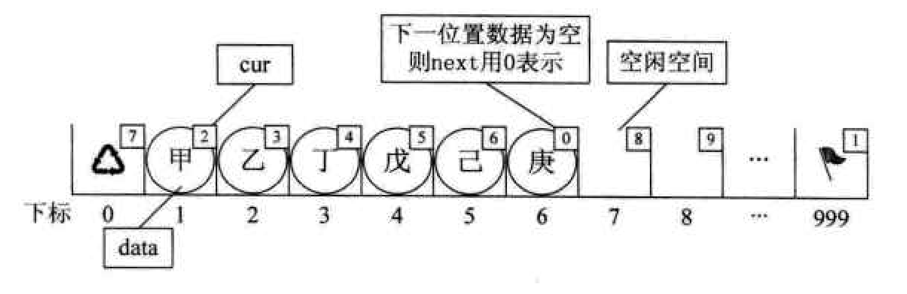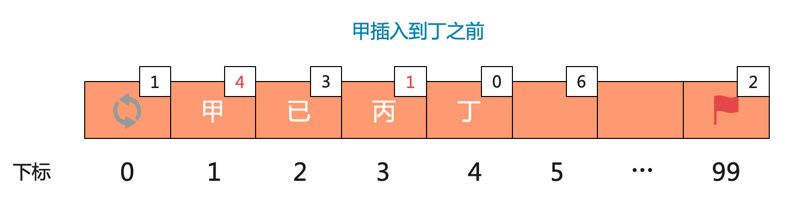## 静态链表

### 静态链表表示法

• 数组的第一个元素的 cur 用来存放备用链表第一个结点的下标
在下图1中，因为整个数组都没有使用过，也就是说整个链表都是备用链表，它存放的 cur 为 1。下图2中，数组的第 7 个元素为空闲空间，所以存放的是 7

• 数组的最后一个元素存放第一个有数值的元素的下标，相当于头结点
在下图1中，因为数组为空，所以为 0；下图2中，第一个有数值的元素为 1，所以为 1

• 静态链表的最后一个元素（非数组的最后元素）的游标为0，表示链尾

• 数组的第一个元素的 cur 为 0 表示链表已满### 静态链表操作

#### 初始化静态链表

``````export default class StaticLinkedList {
constructor() {
this.space = [] //静态链表使用一维数组space来表示
this.length = 0 //链表的长度
this.MAX_SIZE = 0 //申请的空间
}

/**
* 静态链表初始化
* @param {*} maxsize 申请的空间
* @returns
*/
init(maxsize) {
//异常处理，由于数组第一个元素和最后一个元素不存放数据，所以申请的空间长度至少是3或以上
if (maxsize < 3) {
throw new Error('申请的空间至少是3或以上')
}

let i = 0
//因为静态链表最后一个元素不用，所以这里 i < maxsize -1
//数组第一个元素的cur用来存放备用链表第一个节点的下标
//初始化时整个数组都没有被使用过，整个链表都是备用链表，所以space.cur = 1
//其余每项的 cur 都存放它的下一个元素，处理逻辑一样
for (i; i < maxsize - 1; i++) {
this.space.push({
cur: i + 1,
data: null,
})
}
//数组最后一个元素的cur用来存放第一个插入元素的下标，相当于头结点
//因为是一个空链表，所以存放的下标是0
this.space[maxsize - 1] = {
cur: 0,
data: null,
}
//申请的空间
this.MAX_SIZE = maxsize
return true
}
}
``````

#### malloc 和 free

##### malloc``````/**
* 模拟申请内存操作
* 在静态链表中，每次插入数据都是从备用链表上取得第一个结点作为待插入的新结点
* @returns
*/
_malloc_ssl() {
//取出备用链表的第一个元素索引，也就是数组第一个元素的 cur
let i = this.space.cur
//如果 i 不存在，表示链表已满
if (i) {
//由于要拿出一个分量来使用了，所以我们就得把它的下一个分量用来做备用
this.space.cur = this.space[i].cur
}
//返回备用链表第一个结点的下标作为待使用的分量
return i
}
``````
##### free``````/**
* 模拟释放内存
* @param {*} index
* @returns
*/
_free_ssl(index) {
//把下标为0，即存放备用链表第一个元素的cur分量赋值给第index个元素
this.space[index].cur = this.space.cur
//data置为null，表示删除了
this.space[index].data = null
//由于第index个元素释放出来了，所以下标为0的元素的cur为index
this.space.cur = index
}
``````

#### 整表创建

1. 如果表满了，需要设置数组第一个元素的 cur 为 0
2. 设置头结点的 cur 为链表第一个元素的下标，即 1
3. 设置链尾，即最后一个链表元素的 cur 为 0

``````/**
* 链表整表创建，尾插法
* @param {*} n
* @returns
*/
create(n) {
if (n < 0 || n > this.MAX_SIZE - 2) {
return false
}
let i = 0,
j
//循环创建节点
for (i; i < n; i++) {
j = this._malloc_ssl()
this.space[j].data = Number.parseInt(Math.random() * 10 + 1)

//如果创建的是最后一个节点，需要设置 cur 为 0
if (j == n) {
this.space[j].cur = 0
}
this.length++
}
//设置头结点
this.space[this.MAX_SIZE - 1].cur = 1
//如果链表满了，需要设置第一个元素的cur为0
if (this.length == this.MAX_SIZE - 2) {
this.space.cur = 0
}
return true
}
``````

#### 清空链表

``````clear() {
//最后一个元素下标，也就是找到头结点
let cursor = this.MAX_SIZE - 1
//循环清空所有的节点
while (this.space[cursor].cur !== 0) {
this._free_ssl(cursor)
cursor = this.space[cursor.cur]
}
this.length = 0
}
``````

#### 插入操作

`_malloc_ssl`给它分配到了`space`的位置，有两个地方发生了变化：``````//最后一个元素下标，也就是找到头结点
let i = 1, cursor = this.MAX_SIZE - 1
//找到第index元素的前一个元素
for (i; i <= index - 1; i++) {
cursor = this.space[cursor].cur
}
//获得空闲分量的下标，也就是新插入元素的下标
let new_node_index = this._malloc_ssl()

//新元素的 cur 应该指向 index 前一个元素的 cur
this.space[new_node_index].cur = this.space[cursor].cur
//把新元素的下标赋值给 index 前一个元素
this.space[cursor].cur = new_node_index
``````

``````insert(index, elem) {
//异常处理，可以插入的范围：1到最后一个元素+1，即可以插入到表尾
if (index < 1 || index > this.length + 1) {
return false
}
//先找到头结点
let i = 1,
cursor = this.MAX_SIZE - 1,
new_node_index = this._malloc_ssl()

//内存已满，插入不进去
if (!new_node_index) {
return false
}

//插入元素，表长+1
this.space[new_node_index].data = elem
this.length++

//找到第index元素的前一个元素，如果 index 为 1，即插入到表头，它的前一个元素就是头结点了
for (i; i <= index - 1; i++) {
cursor = this.space[cursor].cur
}

//新元素的 cur 应该指向 index 前一个元素的 cur
this.space[new_node_index].cur = this.space[cursor].cur
//把新元素的下标赋值给 index 前一个元素
this.space[cursor].cur = new_node_index

//如果链表满了，需要设置第一个元素的cur为0，表示已满
if (this.length == this.MAX_SIZE - 2) {
this.space.cur = 0
}
}
``````

#### 删除操作``````delete(index) {
//异常处理
if (index < 1 || index > this.length) {
return false
}
//找到 index 的前一个元素
let i = 1,
cursor = this.MAX_SIZE - 1
for (i; i <= index - 1; i++) {
cursor = this.space[cursor].cur
}
//第index个元素的下标
i = this.space[cursor].cur
//前一个元素的cur指向index元素的cur，即表示 index已经删除了，前一个元素指向它后一个元素
this.space[cursor].cur = this.space[i].cur
this._free_ssl(index)
this.length--
return true
}
``````